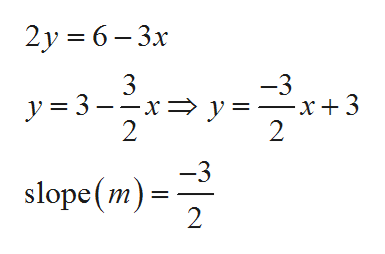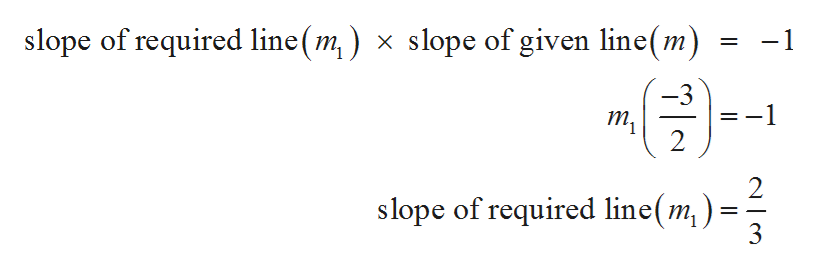# find the equation of the line passing through the point ( 2,3) and perpendicular to the line 2y = 6 - 3x

Question
6 views

find the equation of the line passing through the point ( 2,3) and perpendicular to the line 2y = 6 - 3x

check_circle

Step 1

In the given question it is required to calculate the equation of line passing through (2,3) and perpendicular to the line 2y = 6 – 3x

Since, it is required to find the slope of given line.

If a line is in the form y = mx + c then m is the slope.

So, slope of given line is:help_outlineImage Transcriptionclose2y 6-3x y-3 -3 -x+3 2 3 —х—D у- 2 -3 slope (m) 2 fullscreen
Step 2

If a line is perpendicular to another line then the p...help_outlineImage Transcriptioncloseslope of required line(m,) x slope of given line(m) -1 = -3 т, 2 2 slope of required line(m) 3 fullscreen

### Want to see the full answer?

See Solution

#### Want to see this answer and more?

Solutions are written by subject experts who are available 24/7. Questions are typically answered within 1 hour.*

See Solution
*Response times may vary by subject and question.
Tagged in

### Math